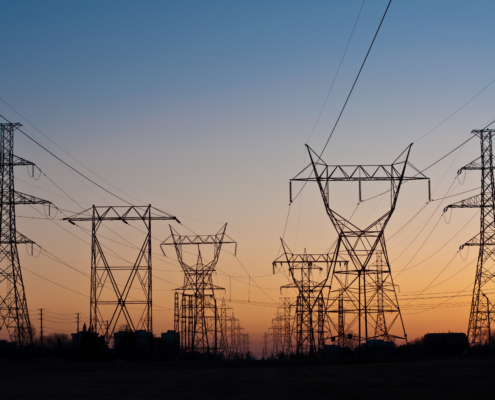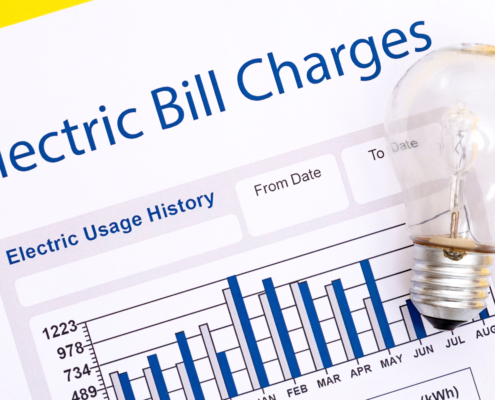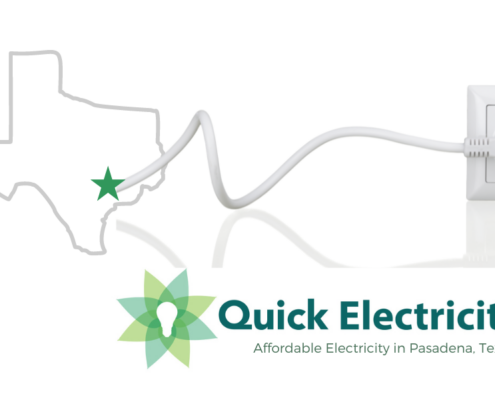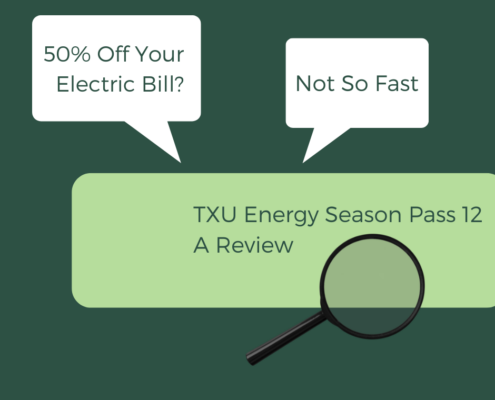Last Updated on December 12, 2022 by Mary Pressler

## Understanding Power Factor Charges in Business Electricity Bills

In a previous article, we discussed how most homes and small businesses are only charged for energy consumption, while larger buildings also pay demand charges. Now we will discuss another charge that is found in many business tariffs – the power factor penalty.

An electric circuit can carry power in any direction, but it normally moves from the voltage supply to the load. However, when you connect electromagnetic devices like motors and transformers, not all the power carried by the circuit is consumed – part of it moves back and forth between the device and the voltage supply.

• When a device uses all the electric power drawn from the voltage supply, it operates at 100% power factor.
• On the other hand, when a device does not consume all the electric power drawn, its power factor is less than 100%.

For example, if you have a lighting system that uses 2,400 watts of power, and you measure a voltage of 120V and a current of 20A, the power factor is 100%. The measured power (2,400W) is equivalent to the apparent power (120V x 20A = 2,400VA)

Now assume that a motor is consuming 1,020W, but you measure a voltage of 240V and a current of 5A. In this case, the apparent power is 1,200VA (240V x 5A), but the real power is only 1,020W. The power factor is calculated by dividing real and apparent power, which results in 0.85 or 85%. However, you still need a circuit that can carry 1,200VA.

Note: To distinguish between apparent power and real power, the first is measured in volt-amperes (VA) and the second is measured in watts (W). Volt-amperes are what you get when multiplying voltage and current directly, hence the name “apparent power”. On the other hand, watts are what you get when power is measured directly. When the power factor is 100%, volt-amperes and watts are equivalent.

## Why Do Electric Companies Penalize Low Power Factor in Buildings?

We explained the concept of power factor with two specific devices, but the power meters used by electric companies can measure PF for an entire building:

• Electric companies establish a minimum PF (for example, 90%), and buildings that operate below this value are penalized.
• Depending on the rate, there may be a compensation for operating above the minimum power factor required.
• As the gap between your power factor and the minimum value increases, the penalty also increases.
• In cases where you are rewarded for a high power factor, the compensation may also increase accordingly.

Note how the motor in the previous example needs a circuit that can carry 1,200VA, even with a power consumption of 1,020W. Well, the same principle applies for electric companies – the grid must have enough capacity to carry the apparent power used by buildings (voltage x current), even when the real power is less. The grid needs larger transformers and power lines to carry the extra current, buildings with low power factor are penalized for this reason. The exact calculation procedure depends on your tariff, but most PF charges have two things in common:

• There is a minimum power factor your building must meet to avoid penalties.
• As the difference between the minimum PF and your building’s PF increases, the penalties also increase.

If your building has a low power factor, you can increase by installing a capacitor bank of the right size. Capacitors can be described as batteries that are designed to charge and discharge many times per second, and they can absorb the power component that moves back and forth between your devices and the voltage supply. This increases the power factor measured by the electric company, and you can reduce or eliminate penalties.

## Understanding Power Factor in Alternating Current Systems

North America uses AC power grids, where the voltage switches from positive to negative at 60 Hz, or 60 times per second. If you connect a power quality analyzer to the voltage supply, you can see a sine wave graph, where voltage and current are constantly switching between positive and negative. Voltage and current switch at the same time in the following example, and when this happens they are considered to be “in phase”:

If you measure power consumption, you will notice it increases and decreases along with voltage and current, but all the power is consumed (100% power factor). In the graph below, you will notice that no electric power is sent back to the grid – it reaches zero watts when voltage and current are zero, but it never drops to negative values.

In this example, the AC voltage is 120V, the AC current is 10A, and the average power is 1,200W. The power factor is 100%, since no power is sent back from the load to the source at any time.

The following graph shows the same voltage and current when the power factor drops to 60%. Note that voltage and current now reach their maximum and zero values at different times, which means they are “out of phase”.

The device consumes power when voltage and current have the same sign, but it sends back power when they have opposite signs. This can be visualized more easily in the following graph:

The apparent power is still 1,200 VA, but the average power consumption is no longer 1,200W. At 60% power factor, it has dropped to 720W. However, you need a circuit that is suitable for 120V and 10A, even when the real power consumption is not 1,200W.

Power factor penalties are used to achieve a higher PF in buildings. Since power lines and transformers must be sized for the apparent power, a low PF makes the grid more expensive. While a single building may not have a considerable impact, a large group of buildings with low PF can be a major burden for the grid.

0 replies

Want to join the discussion?
Feel free to contribute!### Understanding the Roles of an Electricity Company### Power Factor Charges and Commercial Electric Bills### Cheapest Electricity in Pasadena, Texas### TXU Season Pass Electricity Plan/